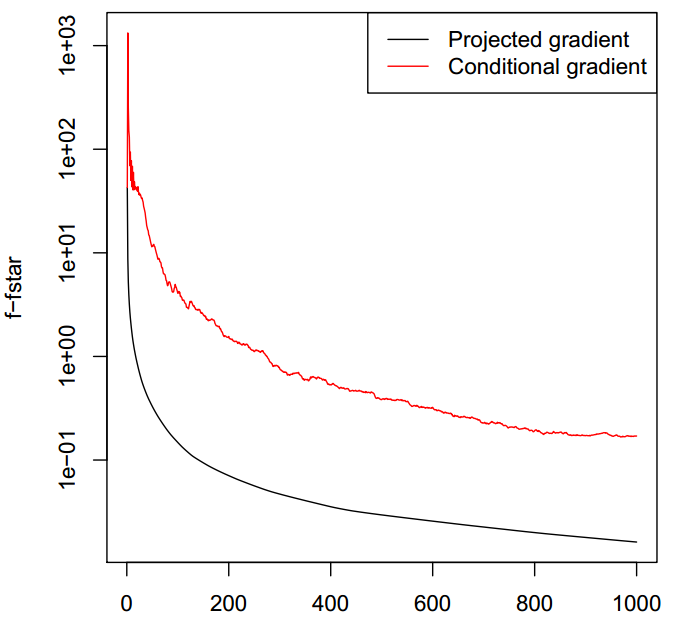# 机器学习中的那些优化算法

## 优化算法の笔记（下） - ADMM & Coordinate Descent

Posted by HC on August 22, 2021

Word转markdown真的好累

# 0x04 Dual Methods and Application

$f^*(y) = \max_{x} y^Tx - f(x)$

1. 对于任意的$x,y$，满足$f(x) + f^{*}(y) \le x^{T}y$
2. 对于任意函数$f$，他的共轭函数的共轭函数，值不大于原函数：$f^{**}(x) \le f(x)$
3. 如果函数$f$是凸的或者凹的，那上面两个性质都可以取等号，并且，满足$x \in \partial f*(y) \leftrightarrow x \in \arg\min _{z} f(z) - y^{T}z$。

$\min_x f(x) \\ s.t. Ax=b$

$\max_u -f^*(-A^Tu) - b^Tu = -g(u) - b^Tu$

Dual subgradient算法就在于处理，我们无法直接求出对偶形式的最大值的时候，我们通过subgradient的方式进行求解，此时对偶问题的subgradient为$∂g(u)-b$。那么接下来顺着subgradient的迭代，我们就可以得到对偶问题的最优值。为了进行下一步的求解，这里是用到了共轭函数具有以下的性质，即

$x \in \partial f^*(y) \leftrightarrow x \in arg\min_z f(z) - y^Tz\\ x \in \partial f^*(-A^Tu) \leftrightarrow x \in arg\min_z f(z) + u^TAz$

1. 首先确定一个初始的$u_{0}$
2. 反复迭代直到满足停止准则
$x^k \in arg\min_x f(x) + (u^{k-1})^TAx\\ u^k = u^{k-1} + t_k (Ax^k - b)$

​ 其中，第一个式子在于找出一个满足subgradient的点，第二步进行迭代更新，$t_{k}$是步长，当$f$函数是严格凸函数的时候，他的共轭函数是可微的，那么次梯度就等于梯度值。当然迭代更新的时候也可以使用proximal subgradient的加速方法。另一方面，dual subgradient的收敛性和primal gradient在本质上是相等的。 ​ 注意，在第一个式子中，我们需要求式子的最小值，这取决于$f$函数的形式是否简单。如果$f$函数是很多个函数的和呢？ 即$f(x)=\sum f_{i}(x)$，此时我们可以考虑小批量的梯度下降，但是，如果$f$函数变成下面这个形式，每个子函数与特定的某部分的x有关。

$f(x) = \sum^B_{i=1} f_i(x_i)$

​ 如果没有等式约束$Ax=b$，那么就可以并行的分块求解，在有约束的情况下，现在我们的优化问题变成下式，即已知变量$x=(x_{1},…,x_{B}) \in R^{n}$，$x_{i} \in R^{n_{i}}$， $\sum_{i=1}^{B} n_{i} =n$，被分割成$B$块，$A=[A_{1}, …, A_{B}]$，$A_{i} \in R^{m* n_{i}}$。

$\min_x \sum_{i=1}^B f_i(x_i) \\ s.t. Ax=\sum_{i=1}^{B} A_ix_i = b$

$x \in \partial f^*(-A^Tu) \leftrightarrow x \in \arg\min_z \sum_{i=1}^B f_i(z_i) + u^TAz \leftrightarrow x_i \in \arg\min_z \sum_{i=1}^B f_i(z_i) + u^TA_iz_i$

$x^k_i \in arg\min_{x_i} f_i(x_i) + (u^{k-1})^TA_ix_i\\ u^k = u^{k-1} + t_k (\sum_{i=1}^B A_ix_i^k - b)$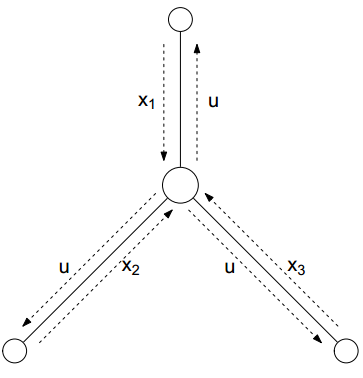$\min_x \sum_{i=1}^B f_i(x_i) \\ s.t. \sum_{i=1}^{B} A_ix_i \le b$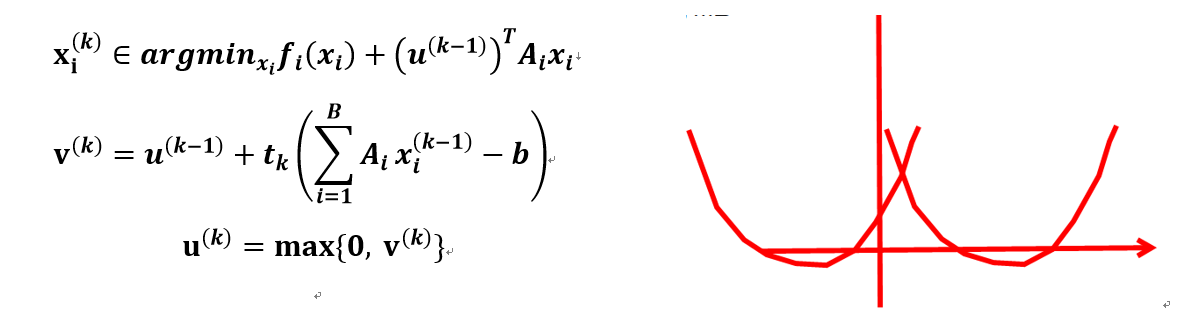​ 这个问题以系统资源占用为例，系统中有$B$个进程，每个进程有自己的决策变量$x_{i}$来决定需要哪些资源，需要多少资源，系统资源的多少限制记为$A$，资源$j$的价格记为$u_{j}$，此时更新过程为下式。如果资源$j$被过度使用，$s_{j}<0$，则提高价格$u_{j}$，反之若资源$j$没咋被使用，就降低$u_{j}$，过程中一直保证资源$j$是非负的。

$u_j^+ = (u_j -ts_j)_+\\ s= b-\sum_{i=1}^B A_ix_i \\ where \quad (a)_+ = max(0,a)$

$\min_x f(x) + \frac{\rho}{2} \|Ax-b\|^2_2\\ s.t. Ax=b$

$x^k \in \arg\min_x f(x) + (u^{k-1})^T Ax + \frac{\rho}{2} \|Ax -b \| ^2_2\\ u^k = u^{k-1} + \rho (Ax^{k-1} - b)$

$0 \in \partial f(x^k) + A^T \left( u^{k-1} + \rho (Ax^k -b) \right) = \partial f(x^k) + A^Tu^k$

​ 这个式子，正好是原问题KKT条件中的stationary condition，这使得在$u^{k}$的迭代中，$x^{k}$也同时慢慢的达到最优，所以较一般的dual subgradient方法来说，具有更好的收敛性，但是由于附加项$\frac{ρ}{2} |Ax-b|_{2}^{2}$，并不具有可分性，所以这个方法不具有dual decomposition。 有没有同时保证收敛和对偶分解的算法呢？答案就是ADMM（Alternating Direction Method of Multipliers）

$\min_x f_1(x_1) + f_2(x_2)\\ s.t. A_1x_1+A_2x_2=b$

$\min_x⁡f_1 (x_1 )+f_2 (x_2 )+\frac{ρ}{2} \|A_1 x_1+A_2 x_2-b\|_2^2\\ s.t. A_1 x_1+A_2 x_2=b$

$L(x_1,x_2,u)=\min_x⁡ f_1 (x_i )+f_2 (x_2 )+\frac{ρ}{2} \|A_1 x_1+A_2 x_2-b\|_2^2+u^T (A_1 x_1+A_2 x_2-b)$

$x_1^{k}=\arg\min_{x_1} L(x_1,x_2^{k-1},u^{k-1} )\\ x_2^{k}=\arg\min_{x_2} L(x_1^k,x_2,u^{k-1} )\\ u^k=u^{k-1}+ρ(A_1 x_1^k+A_2 x_2^k-b)$

$x_1^k=\arg\min_{x_1} f_1 (x_1 )+\frac{ρ}{2} \|A_1 x_1+A_2 x_2^{k-1}-b+w^{k-1} \|_2^2\\ x_2^k=\arg\min_{x_2} f_2 (x_2 )+\frac{ρ}{2} \|A_1 x_1^k+A_2 x_2-b+w^{k-1} \|_2^2\\ w^{k}=w^{k-1}+A_1 x_1^k+A_2 x_2^k-b=w^0+∑_{i=1}^k \left(A_1 x_1^i+A_2 x_2^i-b \right)$

$\min_{\beta} f(\beta) + \lambda \|D\beta\|_1 \rightarrow \min_{\beta} f(\beta) + \lambda \|\alpha\|_1 \quad s.t. D\beta = \alpha$

$β^k=(X^T X+ρD^T D)^+ (X^T y+ρD^T (a^{k-1}-w^{k-1} ))\\ a^k=S_{λ/ρ} (Dβ^k+w^{k-1} )\\ w^k=w^{k-1}+Dβ^k-a^k$

## 0x04_2 Coordinate Descent

$\min f(x) = g(x) + \sum_{i=1}^n h_i(x_i)$

Coordinate Descent运行我们沿着坐标轴一维一维的优化。首先初始化$x^0$，在第k次迭代中执行每个维度的更新

$x_1^k \in \arg\min_{x_1} f(x_1, x_2^{k-1}, ..., x_n^{k-1})\\ x_2^k \in \arg\min_{x_1} f(x_1^k, x_2, ..., x_n^{k-1})\\ x_n^k \in \arg\min_{x_1} f(x_1^k, x_2^k, ..., x_n)$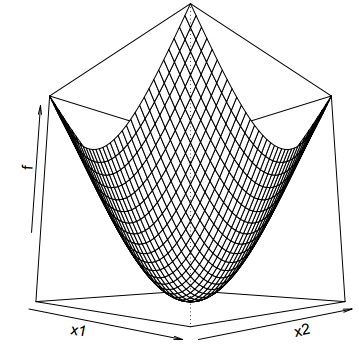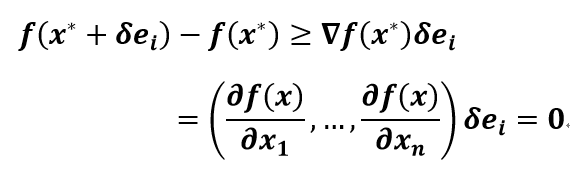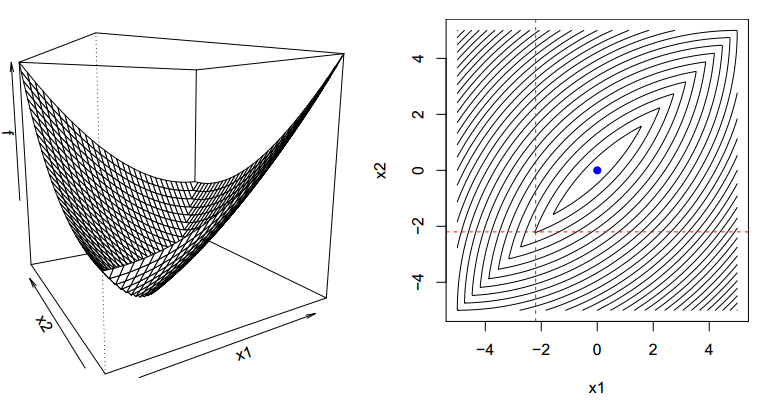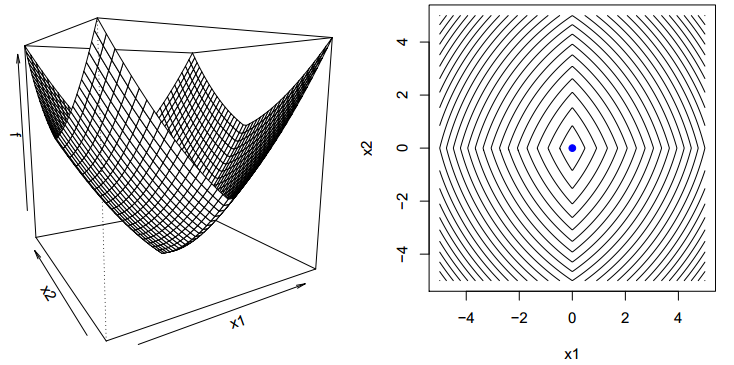### Linear Regression

$\min_{\beta} \frac{1}{2} \| y-X\beta\|_2^2$

$0=∇_i f(β)=X_i^T (Xβ-y)=X_i^T (X_i β_i+X_{-i} β_{-i}-y) → β_i=\frac{X_i^T (y -X_{-i} β_{-i} )}{X_i^T X_i}$### SVM (SMO算法)

$\min_{\beta,\beta_0,\delta} \frac{1}{2} \|\beta\|_2^2 + C \sum_{i=1}^n \delta_{i} \\ s.t. \quad -\delta_i \le 0,i=1,2,...,n\\ \quad 1-\delta_i-y_i(x_i^T\beta + \beta_0) \le 0, i=1,2,...,n$

$\max_{w} -\frac{1}{2}W^T\hat{X}\hat{X}^TW + W^T1 \\ s.t. \quad 0\le w_i \le C, i=1,2,...,n\\ \quad W^Ty=0$

Stationary：$\sum_{i=1}^{n}w_{i}y_{i}=0, C-v_{i}-w_{i}=0, \beta=\hat{X}^{T}W=\sum_{i=1}^{n}w_{i}x_{i}y_{i}$

Complementary：$v_{i}\delta_{i}=0, w_{i}(1-\delta_{i}-y_{i}(x_{i}^{T}\beta+\beta_{0}))$

$w_iy_i = -\sum_{k \neq i}^n w_ky_k$

SMO启发式的选择两个block $w_{1}$和$w_{2}$同时更新，此时有

$w_1=\left( -\sum_{k=3}w_ky_k - w_2y_2 \right) \frac{1}{y_1} = (\pi - w_2y_2) \frac{1}{y_1} \\ \rightarrow w_2y_2 + w_1y_1 = \pi$$x^k=P_C \left( x^{k-1} - t_k \triangledown f(x^{k-1}) \right)$

$x^k=P_C \left( \arg\min_y \triangledown f(x^{k-1}) ^T (y-x^{k-1}) + \frac{1}{2t} \| y - x^{k-1} \|_2^2 \right)$

conditional gradient method（或者叫Frank-Wolfe Method）采用的也是对$f$的局部的线性展开的性质，其展开的形式如下，其中$S$表示常数。

$f^{linear}(s) = f(x^{k-1}) + \triangledown f(x^{k-1})^T (s-x^{k-1}) \\ =\triangledown f(x^{k-1})^T s + S$

Conditional gradient通过最小化这个线性近似来找下一个迭代点，可以看到，如果问题本身是无约束优化问题，那么$f^{linear} (s)$的最小值就是负无穷，所以conditional gradient并不适用于无约束优化问题。此时他的迭代式子为

$s^{k-1} \in \arg\min_{s \in C} \triangledown f(x^{k-1})^T s \\ x^k = (1-\gamma_k) x^{k-1} + \gamma_k s^{k-1} = x^{k-1} + \gamma_k (s^{k-1} - x^{k-1})$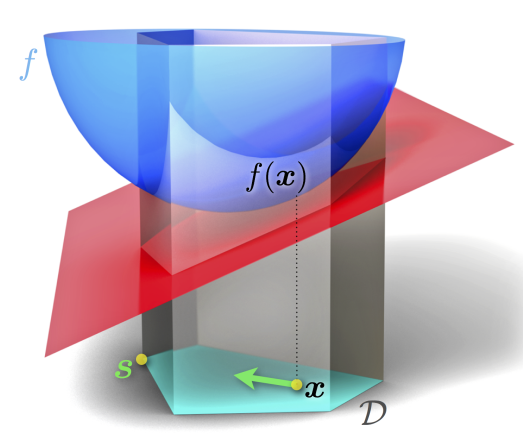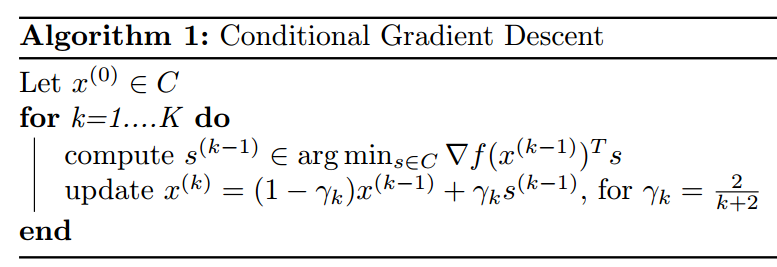$s^{k-1} \in \arg\min_{ \|s\| \le t} \triangledown f(x^{k-1})^T s$

$s^{k-1} \in \arg\min_{ \|At\| \le t} \triangledown f(x^{k-1})^T At\\ = -t \left( \arg\max_{ \|A\| \le 1} \triangledown f(x^{k-1})^T A\right)$

$\min_x f(x) \\ s.t. \|x\| \le t$

$s^{k-1} \in -t \partial\| \triangledown f(x^{k-1})\|_{\infty}$

$i_{k-1} \in \arg\min abs(\triangledown_i f(x^{k-1}))\\ x^k = (1-\gamma_k)x^{k-1} - t \gamma_k sign\left( \triangledown_{i_{k-1}} f(x^{k-1}) \right) e_{i_{k-1}}$